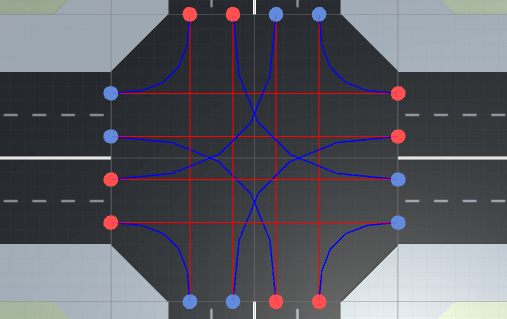# Everything about interpolation in Unity with C# code

## Introduction

According to Wikipedia, interpolation is a method of constructing new data points within the range of a discrete set of known data points. The easiest form of interpolation is linear interpolation, where you follow a straight line from A to B (the known data points).

When interpolating you are using a parameter called t, which is defined as 0 when you are at the start position and 1 if you are at the end position. So to interpolate linearly from A to B we get the following function: f(t) = (1 - t) * A + t * B. If you insert t = 0, you get f(0) = A, which is the start position. Unity has this function built-in, so if you write Vector3.Lerp(A, B, t), it's the same as writing (1 - t) * A + t * B.

But following straight lines are kinda boring - it's more fun to follow curves. The curves are here called splines, which is "a numeric function that is piecewise-defined by polynomial functions, and which possesses a high degree of smoothness at the places where the polynomial pieces connect." The most common splines are quadratic, with one control point, and cubic, with two control points. These control points are determining the shape of the spline.

A Cubic Hermite spline is a cubic polynomial spline. One example of these is the Catmull-Rom spline. But these can also be specified in other ways, where the Bézier form being the most common. However, these two methods provide the same set of splines, and data can be easily converted between the Bézier and Hermite forms, so the names are often used as if they were synonymous.

This tutorial is divided into the following sections:

...and when you are done you will be able to guide your self-driving cars through a traffic intersection: